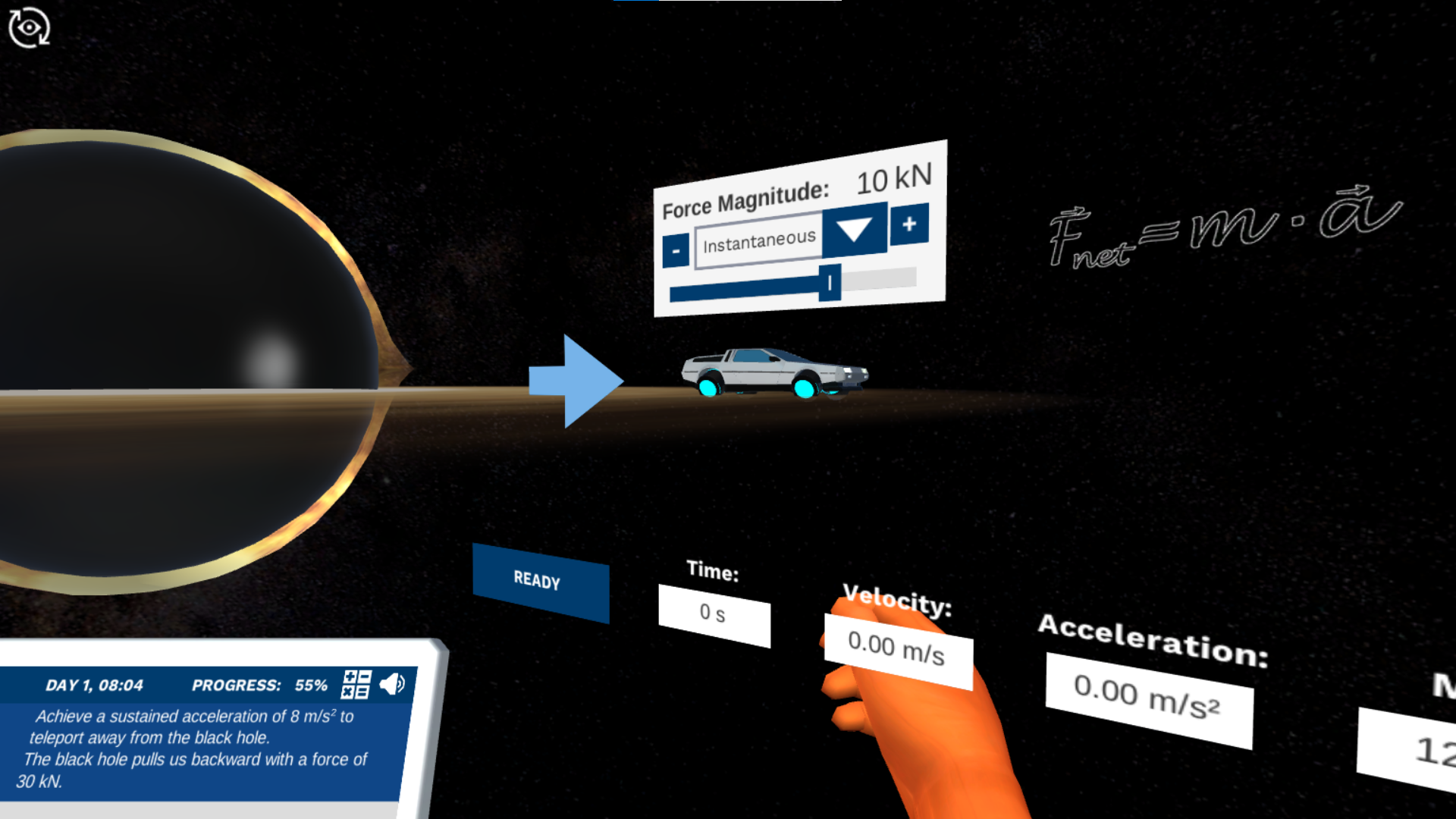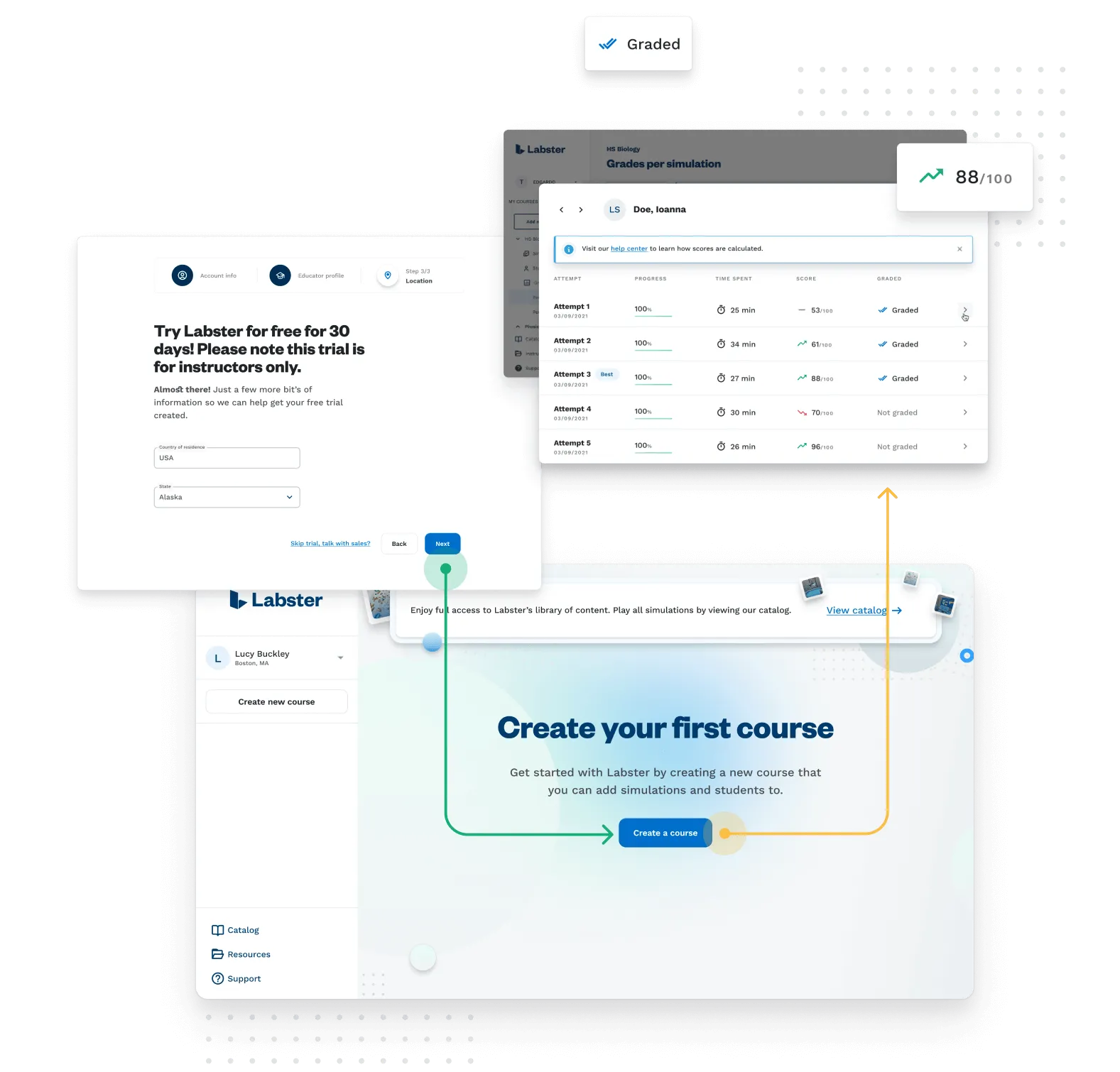Back to simulations

# Newton's Second Law of Motion: Speed and Acceleration Virtual Lab

Travel through time and space to help Newton remember his second law. Use this physically realistic simulation to experiment with forces and masses, and observe their effects on acceleration and velocity.High SchoolNewton's second law of motion describes the true power of forces. In this simulation, you will learn about Newton's Second Law: the relationship between the forces that act on a body, that body's mass, and its acceleration.

Apply forces and observe the resulting motion

In this sim, you will help Newton regain his memory of his second law of motion by applying forces on a body with adjustable mass to control its acceleration and produce different kinds of motion. Includes experimentation tasks and directed challenges that require the student to take the effects of added forces into account or produce a specific motion.

Relate force, mass, and acceleration

This simulation builds the student's understanding of the relationship between forces mass and acceleration with progressively incrementing complexity. By the end, the student is capable of fully understanding the impact of a force on a body's motion, and they can use and rearrange equations to relate force, mass, and acceleration.

To this end, we present the student with a variety of interactive physical scenarios (including scenarios with and without friction and with and without additional forces to balance) and plenty of opportunities to play with the physical parameters (including constant and instantaneous forces, a wide range of force magnitudes, exchangeable masses and friction surfaces.) well integrated into a motivating narrative.

Rediscover Newton’s second law of motion

In the end, you will have rediscovered Newton's second law of motion alongside him in all of its nuance. Will you be able to unlock the power of forces?

#### Learning Objectives

At the end of this simulation, you will be able to:

• Use and rearrange equations to relate force, mass, and acceleration

• Calculate how changing the magnitude of balanced and unbalanced forces impacts the speed and acceleration of a body

• Interpret speed from a distance/time graph

#### Techniques in Lab

At the end of this simulation, you will be able to:

• Newton’s second law of motion
• Acceleration
• Velocity
• Mass
• Friction
• Vectors
• Vector sum
• Dynamics
• Motion
• Force
• Net force

## How do virtual labs work?

Engage students in science through interactive learning scenarios. Simulate experiments, train lab techniques, and teach theory through visual experiences that enhance long-term learning outcomes.300+ Web-based simulations that can be played on laptops, Chromebooks, and tablets/iPads without installing any softwareTeacher dashboard to automate grading and track student progressEmbedded quizzes to help students master science contentLibrary of learning resources, lab reports, videos, theory pages, graphics and more

### Get Started Now!

Try Labster with your students right away.Track student progressAssess with embedded quiz questionsInvite your students to play simulationsExplore over 300 Labster simulations